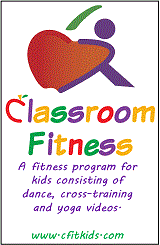Monday, June 17th 2019
Welcome GuestNumber and Number Sense
1.1a Count/Write 0-100
1.1b Tens and Ones- Place Value
1.2 Skip Count (2, 5, 10)
1.3 Identify/represent halves, thirds, fourths
Computation and Estimation
1.4 Reasonable Magnitude
1.6 Create and Solve Story Problems
1.7 Coin values to total 100 cents
1.8 Tell time to half hour
1.9 Nonstandard length, weight, volume
1.10a Compare volumes
1.10b Compare weights
1.11 Calendar language
1.12 Identify, describe, sort figures
1.13 Figures in the environment
Probability and Statistics
1.14 Data: tables, picture and object graphs
1.15 Interpret information from graph
Patterns, Functions, and Algebra
1.16 Sort/classify-color, size, thickness, shape
1.17 Recognize, describe, extend, create patterns
1.18 Understand equality through the use of the = sign
Number and Number Sense
2.1a Read, write, identify place value (3 digits)
2.1b Round 2-digit numbers
2.1c Compare numbers
2.3c Compare unit fractions
2.4 Counting forward and backward, even/odd
2.2 Ordinal positions 1st to 20th, write ordinal numbers
2.3 a, b Fractions: halves, thirds, fourths, sixths, eighths
Computation and Estimation
2.5 Recall addition/subtraction facts to 20
2.6 Estimate and find sums
2.7 Estimate and find differences
2.8 Solve one- and 2-step and - problems
2.9 Related facts
2.11a Length: cm and inch
2.11c Liquid volume
2.14 Temperature
2.16 Plane and solid figures
Probability and Statistics
2.18 Use data to predict outcomes
2.19 Analyze data in graphs
Patterns, Functions, and Algebra
2.21 Solve problems by completing numerical sentences
2.22 Understand equality
Number and Number Sense
3.1a Place Value- 6 digits
3.1b Rouding- 4 digits
3.1c Comparing Numbers- 4 digits
3.2 Inverse Relationships
3.3 Fractions and Mixed Numbers
Computation and Estimation
3.5 Multiplication and Division Facts
3.6 Represent Multiplication and Divison
3.9 US Customary/Metric Units to Measure
3.10 Area/Perimeter
3.11 Telling Time/Elapsed Time
3.12 Equivalent Periods of Time
3.13 Temperature
3.14 Plane/Solid Geometric Figures
3.15 Points, Lines, and Angles
3.16 Congruence
Probability and Statistics
3.17 Organize and Contruct Graphs
3.18 Probability
Patterns, Functions, and Algebra
3.20 Properties/Equality
Basic Facts Practice
Multiplication/Division
Number and Number Sense
4.1 Seven Digit Numbers
4.3 Decimals to Thousandths
Computation and Estimation
4.4a Estimation- All operations
4.4b Whole Number Operations
4.4c Whole Number Computation- Division
4.4d Single and Multi Step Word Problems
4.5a Greatest Common Factor and Least Common Multiple
4.5d Solve Problems with Fractions and Decimals
4.8 Liquid Volume
4.9 Elapsed Time
4.10 Points, lines, line segments, rays, angles
4.11 Translation, reflection, rotation
Probability and Statistics
4.13 Probability
4.14 Line and bar graphs
Patterns, Functions, and Algebra
4.15 Numerical and geometric patterns
4.16 Equality/Associative Property
Basic Facts Practice
Multiplication/Division
Number and Number Sense
5.1 Rounding decimals
5.2 Fractions/Decimals
5.3 Prime/Composite and Odd/Even
Computation and Estimation
5.5 Compuation of Decimals
5.4 Whole Number Problems , -, x, /
5.6 Fractions , -
5.7 Order of Operations
5.8 Perimeter, Volume, Area, Temperature
5.10 Elapsed Time
5.11 Angle Measures
5.13 Plane Figures
5.12 Classify angles/triangles
Probability and Statistics
5.14 Probability Sample Space
5.15 Stem and Leaf
5.16 Mean, Median, Mode, Range
Patterns, Functions, and Algebra
5.18 Variables and Equations
5.19 Distributive Property
Basic Fact Practice